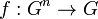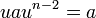# Characterization of subgroup of neutral elements of reducible multiary group

## Statement

Consider a reducible multiary group, i.e., a$n$-ary group$G$ with multiplication$f:G^n \to G$ such that there exists a group structure on$G$ such that$f(a_1,a_2,\dots,a_n) = a_1a_2\dots a_n$ for all (possibly repeated)$a_1,a_2,\dot,a_n \in G$, with the multiplication on the right being as per the group structure.

Let$C$ be the subset of$G$ comprising those elements that are neutral for$f$. Consider$C$ as a subset of$G$ with its (ordinary 2-ary) group operation. The claim is that$C$ is a subgroup of$G$, and in fact, it is the characteristic subgroup comprising those elements in the center of$G$ whose order divides$n - 1$.

## Note

Note that due to the equivalence of definitions of reducible multiary group, we know that neutral elements exist if and only if the$n$-ary group is reducible. Thus, for irreducible$n$-ary groups, we would get an empty set instead of a subgroup.

## Proof

Given: Group$G$ with identity element$e$.$C$ is the subset of$G$ comprising those elements$u$ of$G$ for which$u^iau^{n-1-i} = a$ for all$a \in G$ and all$i \in \{ 0,1,\dots, n-1\}$.

To prove:$C$ is precisely the subgroup of$G$ comprising the elements$u$ in the center of$G$ with$u^{n-1} = e$.

Proof:

Step no. Assertion/construction Explanation
1 Every element of$C$ is in the center of$G$. Set$i = 0$ to get$au^{n-1} = a$. Now set$i = 1$ to get$uau^{n-2} = a$. Equating the two left sides gives$au^{n-1} = uau^{n-2}$. Cancel$u^{n-2}$ from the right of both expressions to get$au = ua$ for all$a \in G$.
2 For all$u \in C$,$u^{n-1} = e$. Set$i = 0$ and$a = e$ to get$eu^{n-1} = e$ which simplifies to$u^{n-1} = e$.
3 If$u$ is in the center of$G$ and$u^{n-1} = e$, then$u \in C$. Consider the product$u^iau^{n-1-i}$. Because$u$ is central, we can commute it past$a$ and get$u^{n-1}a$, which simplifies to$a$ since$u^{n-1} = e$. This is true for all$a \in G$ and all$i \in \{0,1,\dots,n-1\}$. Thus,$u \in C$.
4 The set of elements$u$ such that$u$ is in the center of$G$ and$u^{n-1} = e$ forms a characteristic subgroup of$G$. The center is already a subgroup, so we need only check that the condition$u^{n-1} = e$ is a subgroup of it. Since abelian implies universal power map is endomorphism, it can be described as the kernel of the$(n-1)$-power map, which is a subgroup. It is characteristic because of the unique manner of definition.
5 We are done Steps (1) and (2) show one direction, Step (3) shows the reverse direction. Step (4) confirms that it is a subgroup.